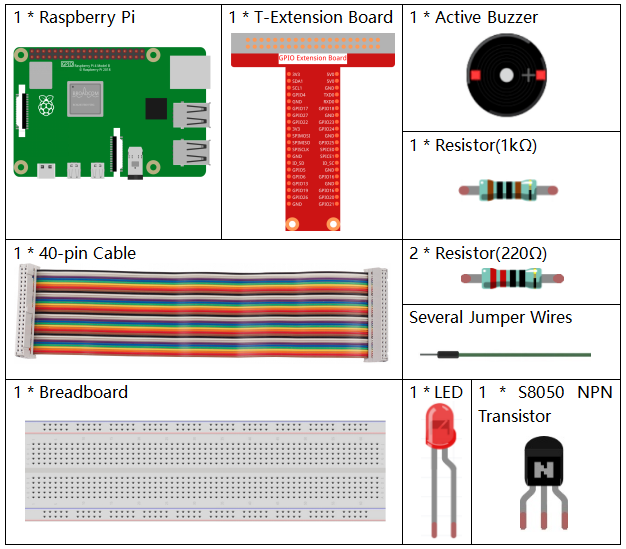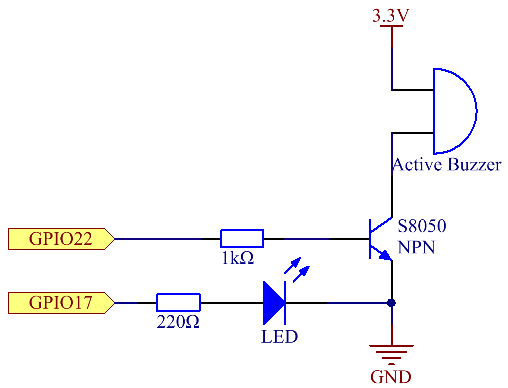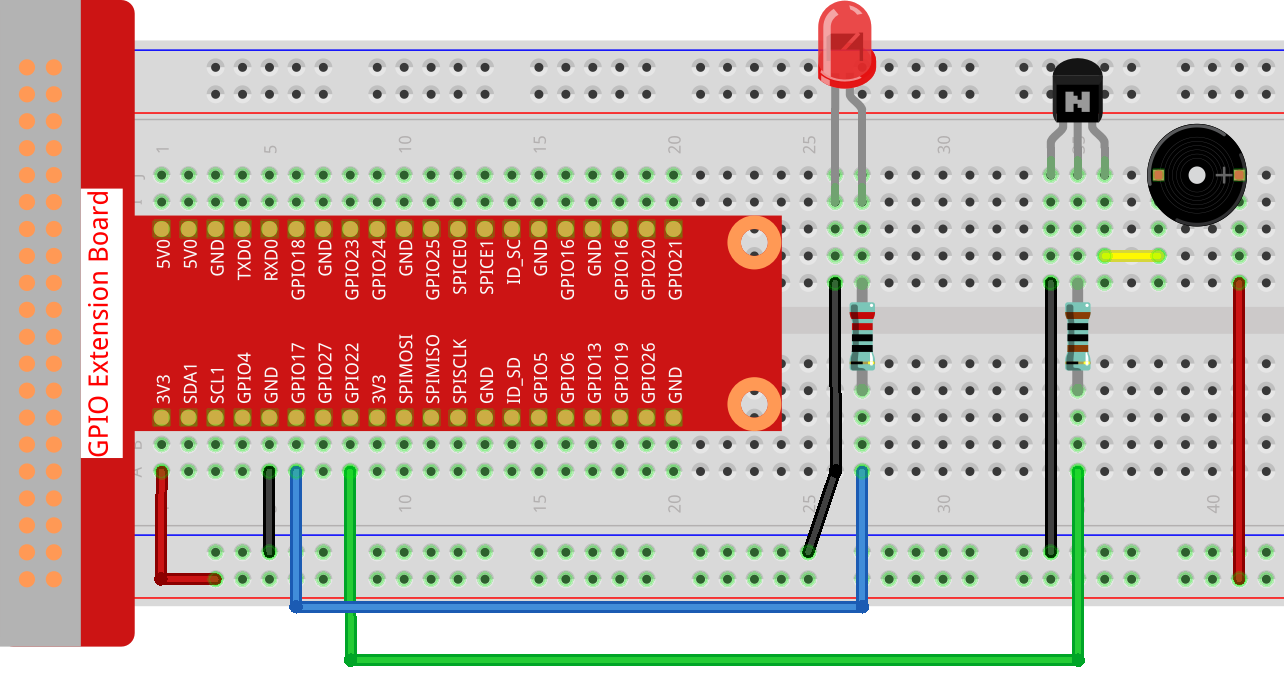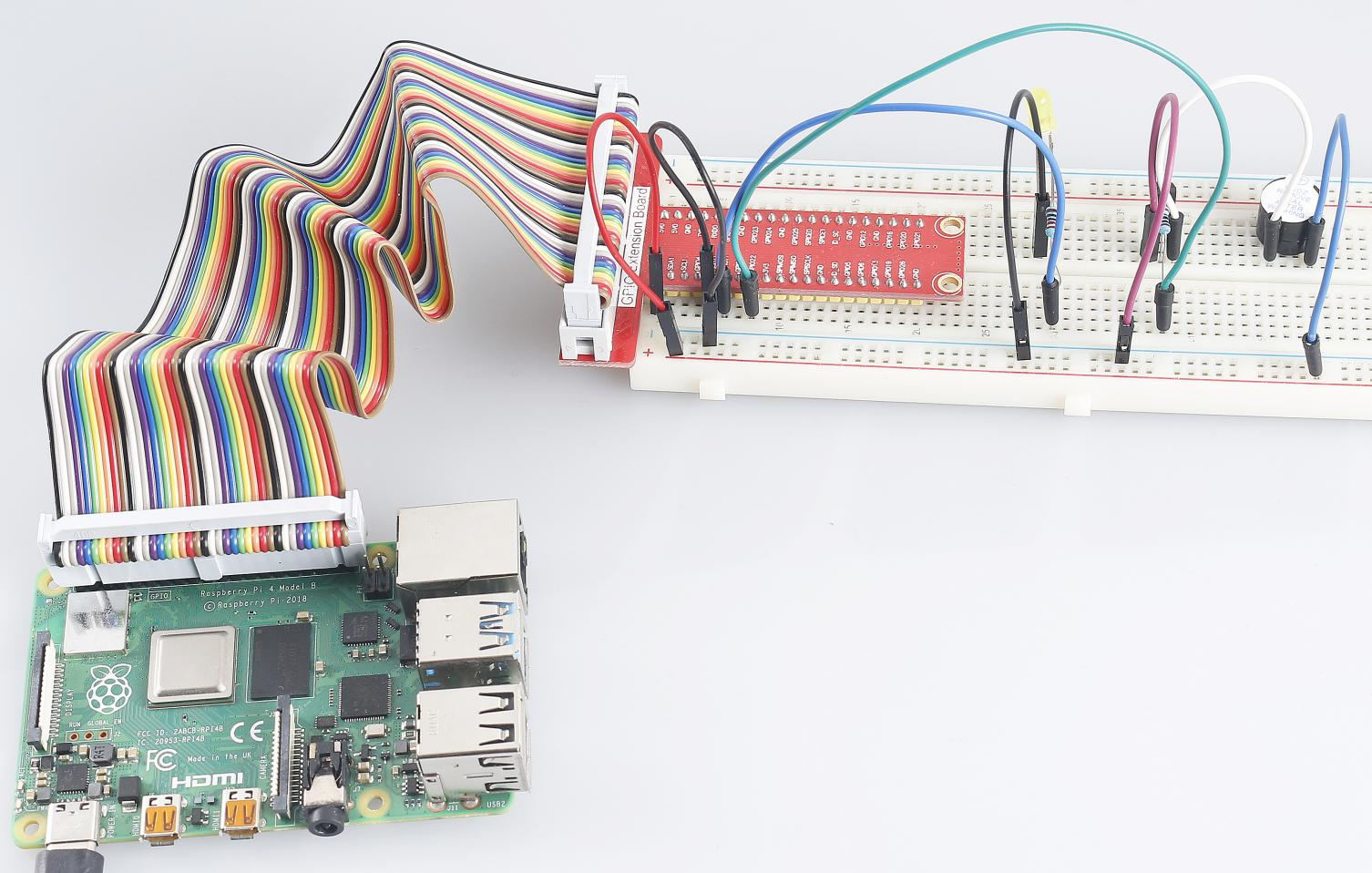# 4.1.16 Morse Code Generator¶

## Introduction¶

In this project, we’ll make a Morse code generator, where you type in a series of English letters in the Raspberry Pi to make it appear as Morse code.

## Required Components¶

In this project, we need the following components.Name

ITEMS IN THIS KIT

Raphael Kit

337

Raphael Kit

COMPONENT INTRODUCTION

GPIO Extension Board

Jumper Wires

Resistor

LED

Buzzer

-

Transistor

## Schematic Diagram¶

 T-Board Name physical wiringPi BCM GPIO17 Pin 11 0 17 GPIO22 Pin 15 3 22## Experimental Procedures¶

Step 1: Build the circuit. (Pay attention to poles of the buzzer: The one with + label is the positive pole and the other is the negative.)Step 2: Open the code file.

```cd ~/raphael-kit/python
```

Step 3: Run.

```sudo python3 4.1.16_MorseCodeGenerator.py
```

After the program runs, type a series of characters, and the buzzer and the LED will send the corresponding Morse code signals.

Code

```#!/usr/bin/env python3
import RPi.GPIO as GPIO
import time

BeepPin=22
ALedPin=17

MORSECODE = {
'A':'01', 'B':'1000', 'C':'1010', 'D':'100', 'E':'0', 'F':'0010', 'G':'110',
'H':'0000', 'I':'00', 'J':'0111', 'K':'101', 'L':'0100', 'M':'11', 'N':'10',
'O':'111', 'P':'0110', 'Q':'1101', 'R':'010', 'S':'000', 'T':'1',
'U':'001', 'V':'0001', 'W':'011', 'X':'1001', 'Y':'1011', 'Z':'1100',
'1':'01111', '2':'00111', '3':'00011', '4':'00001', '5':'00000',
'6':'10000', '7':'11000', '8':'11100', '9':'11110', '0':'11111',
'?':'001100', '/':'10010', ',':'110011', '.':'010101', ';':'101010',
'!':'101011', '@':'011010', ':':'111000',
}

def setup():
GPIO.setmode(GPIO.BCM)
GPIO.setup(BeepPin, GPIO.OUT, initial=GPIO.LOW)
GPIO.setup(ALedPin,GPIO.OUT,initial=GPIO.LOW)

def on():
GPIO.output(BeepPin, 1)
GPIO.output(ALedPin, 1)

def off():
GPIO.output(BeepPin, 0)
GPIO.output(ALedPin, 0)

def beep(dt):       # dt for delay time.
on()
time.sleep(dt)
off()
time.sleep(dt)

def morsecode(code):
pause = 0.25
for letter in code:
for tap in MORSECODE[letter]:
if tap == '0':
beep(pause/2)
if tap == '1':
beep(pause)
time.sleep(pause)

def main():
while True:
code = code.upper()
print(code)
morsecode(code)

def destroy():
print("")
GPIO.output(BeepPin, GPIO.LOW)
GPIO.output(ALedPin, GPIO.LOW)
GPIO.cleanup()

if __name__ == '__main__':
setup()
try:
main()
except KeyboardInterrupt:
destroy()
```

Code Explanation

```MORSECODE = {
'A':'01', 'B':'1000', 'C':'1010', 'D':'100', 'E':'0', 'F':'0010', 'G':'110',
'H':'0000', 'I':'00', 'J':'0111', 'K':'101', 'L':'0100', 'M':'11', 'N':'10',
'O':'111', 'P':'0110', 'Q':'1101', 'R':'010', 'S':'000', 'T':'1',
'U':'001', 'V':'0001', 'W':'011', 'X':'1001', 'Y':'1011', 'Z':'1100',
'1':'01111', '2':'00111', '3':'00011', '4':'00001', '5':'00000',
'6':'10000', '7':'11000', '8':'11100', '9':'11110', '0':'11111',
'?':'001100', '/':'10010', ',':'110011', '.':'010101', ';':'101010',
'!':'101011', '@':'011010', ':':'111000',
}
```

This structure MORSE is the dictionary of the Morse code, containing characters A-Z, numbers 0-9 and marks “?” “/” “:” “,” “.” “;” “!” “@” .

```def on():
GPIO.output(BeepPin, 1)
GPIO.output(ALedPin, 1)
```

The function `on()` starts the buzzer and the LED.

```def off():
GPIO.output(BeepPin, 0)
GPIO.output(ALedPin, 0)
```

The function `off()` is used to turn off the buzzer and the LED.

```def beep(dt):   # x for dalay time.
on()
time.sleep(dt)
off()
time.sleep(dt)
```

Define a function `beep()` to make the buzzer and the LED emit sounds and blink in a certain interval of `dt`.

```def morsecode(code):
pause = 0.25
for letter in code:
for tap in MORSECODE[letter]:
if tap == '0':
beep(pause/2)
if tap == '1':
beep(pause)
time.sleep(pause)
```

The function `morsecode()` is used to process the Morse code of input characters by making the “1” of the code keep emitting sounds or lights and the “0”shortly emit sounds or lights, ex., input “SOS”, and there will be a signal containing three short three long and then three short segments “ · · · - - - · · · ”.

```def main():
while True:
When you type the relevant characters with the keyboard, `upper()` will convert the input letters to their capital form.
`printf()` then prints the clear text on the computer screen, and the `morsecod()` function causes the buzzer and the LED to emit Morse code.